CAT  >  Practice Questions Level 2: Functions - 2

# Practice Questions Level 2: Functions - 2 - Notes | Study Level-wise Practice Questions for CAT Preparation - CAT

 1 Crore+ students have signed up on EduRev. Have you?

This EduRev document offers 20 Multiple Choice Questions (MCQs) from the topic Functions (Level - 2). These questions are of Level - 2 difficulty and will assist you in the preparation of CAT & other MBA exams. You can practice/attempt these CAT Multiple Choice Questions (MCQs) and check the explanations for a better understanding of the topic.

Question for Practice Questions Level 2: Functions - 2
Try yourself:If f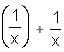f(-x) = 2x, what is the value of f(2)?

Question for Practice Questions Level 2: Functions - 2
Try yourself:Let f(x) = 7x3 + 23x + 18. If the value of f(x + 8) - f(x + 7) - f(x + 6) + f(x + 5) - f(x + 4) + f(x + 3) + f(x + 2) - f(x + 1) is a constant, then find that value.

Question for Practice Questions Level 2: Functions - 2
Try yourself:Let f(x) = x5 + ax4 + bx3 + cx2 + dx + e and f(1) = f(2) = f(3) = f(4) = f(5). Then a is equal to

Question for Practice Questions Level 2: Functions - 2
Try yourself:If f(x) = (x5 - 1) (x3 + 1), g(x) = (x2 - 1) (x2 - x + 1) and h(x) is a polynomial such that f(x) = g(x)h(x), what is the value of h(1)?

Question for Practice Questions Level 2: Functions - 2
Try yourself:Consider a recursive function C defined by:
C(n, 0) = n + 1
C(0, i) = C(1, i - 1) for i > 0
C(n, i) = C(C(n -1, i), ( i - 1)) for n > 0 and i > 0
Compute C(1, 2) from the above definition.

Question for Practice Questions Level 2: Functions - 2
Try yourself:Let f and g be functions and f(g(x)) = x + 2 and f(s) =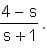. What is the value of g(t)?

Question for Practice Questions Level 2: Functions - 2
Try yourself:Let f(x) = 1 -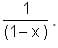Find f(f(f(…f(4)..))), when there are 1997 f's in the composition.

Question for Practice Questions Level 2: Functions - 2
Try yourself:The value of the function f(n) is 35/16 which is represented as f(n) =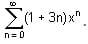Find the value of x(Answer upto one decimal place).

Question for Practice Questions Level 2: Functions - 2
Try yourself:If f(x + y) = f(x) + f(y) and f(1) + f(2) + f(3) … f(10) = 1, find the value of f(1).

Question for Practice Questions Level 2: Functions - 2
Try yourself:If f is a function such that f(0) = 2, f(1) = 3 and f(x + 2) = 2f(x) - f(x + 1), then f(5) is equal to

Question for Practice Questions Level 2: Functions - 2
Try yourself:If the graph of y = f(x) is transformed to the graph of 2y - 6 = -4f(x - 3), point (a, b) on the graph of y = f(x) becomes point (A, B) on the graph of 2y - 6 = -4f(x - 3), where A and B are given by:

Question for Practice Questions Level 2: Functions - 2
Try yourself:Given below is a graph made up of line segments shown as thick lines. Which of the following options is correct?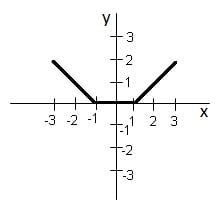Question for Practice Questions Level 2: Functions - 2
Try yourself:The graphs of f(x) and g(x) are shown below: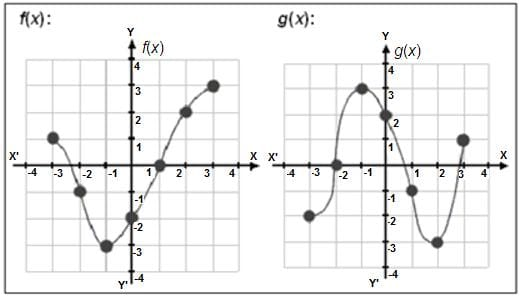Find (fog)(-1).

Question for Practice Questions Level 2: Functions - 2
Try yourself:A sphere's radius is increasing at the rate of 4.2 cm/sec. Find the rate at which its area of surface changes when the radius is 100 cm.

Question for Practice Questions Level 2: Functions - 2
Try yourself:A cube's edge is changing at the rate of 2.61 cm/sec. The rate of change of its longest diagonal will be (approx.)

Question for Practice Questions Level 2: Functions - 2
Try yourself:A large company intends to maximise its profits using calculus. Its unit sales price is Rs. 500 and cost of production = 100 + 5X2, where X is the total units sold or produced. Find the most profitable production level.

Question for Practice Questions Level 2: Functions - 2
Try yourself:A company sells its products at Rs. 155 per kg and its cost of manufacture is given by Rs. (X2 + 5) per kg. Find the production level for maximum profit, if X = production level.

Question for Practice Questions Level 2: Functions - 2
Try yourself:In Indore, Annapurna area has 2000 telephone subscribers and government collects fixed charges of Rs. 280 per month from each subscriber. The government proposes to increase the tariff and if it is forecasted for each rupee increase, there will be an equivalent amount of subscribers discontinuing. So, what increase will bring maximum revenue to the government?

Question for Practice Questions Level 2: Functions - 2
Try yourself:The maximum area of a rectangle with perimeter equal to 12 units will be

Question for Practice Questions Level 2: Functions - 2
Try yourself:Let f1(n) = n - 28  n  6
f2(m) = m - 16 ≤ m  - 1
f(x) = f(m) + f(n)

What is the value of fmax(x) and fmin(x)?

The document Practice Questions Level 2: Functions - 2 - Notes | Study Level-wise Practice Questions for CAT Preparation - CAT is a part of the CAT Course Level-wise Practice Questions for CAT Preparation.
All you need of CAT at this link: CAT

## Level-wise Practice Questions for CAT Preparation

277 docs
 Use Code STAYHOME200 and get INR 200 additional OFF

## Level-wise Practice Questions for CAT Preparation

277 docs

### How to Prepare for CAT

Read our guide to prepare for CAT which is created by Toppers & the best Teachers

Track your progress, build streaks, highlight & save important lessons and more!

,

,

,

,

,

,

,

,

,

,

,

,

,

,

,

,

,

,

,

,

,

;